|

# 连在墙上钉东西的勇气都没有,你的房子是租的吧!

最近有小伙伴问东亭，装修结束以后搬进新房，如果想要在墙上挂幅画，或者是挂杆怎么办？要用什么钉子，怎么样才能最少的破坏墙面呢？

作为女孩子，东亭非常理解这种刚装修完，总觉得家里的大白墙空落落的，一旦看到好看的软装小物比如挂画、相框就会心痒痒的，想要赶快把它们带回家里好好布置的心情。▲ 图源：oraclefox.com

而且除了软装外，入住之后，小户型总是需要绞尽脑汁去思考怎么收纳能够更省空间，那么这时候收纳上墙就会是很好的选择。

所以这些都绕不开在墙上钉钉子的步骤。

一

墙上挂画怎么做

▏挂画神器无痕钉▕

如果小伙伴们想要在墙上悬挂的东西是相框挂画之类的话，那就不要纠结了，可以选择挂画神器无痕挂钩。

虽然叫挂钩，但实际上它也是一种钉子，常见的有三钉和四钉。

和直接在墙上钉钉子相比，无痕钉对墙面的破坏性更小，操作更简单，一把小钉锤就能搞定，女生也可以轻松安装。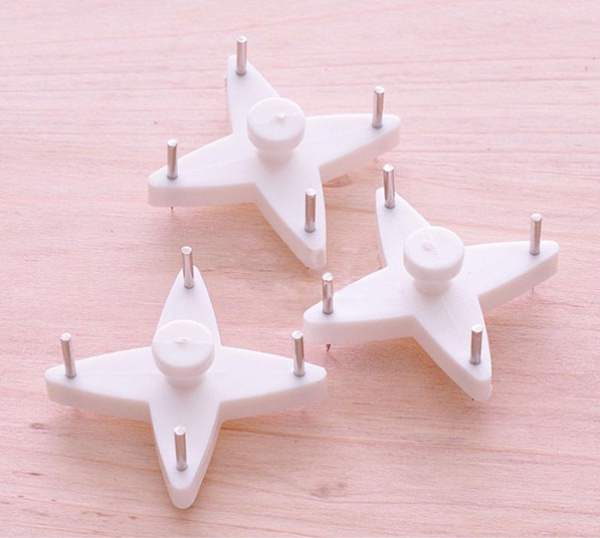▲ 图源： meilishuo.com

它的面积不大，但是有多种型号和样式可以选择。

购买时，小伙伴们需要了解的是相框背面的吊钩样式，来选择合适的无痕钉类型。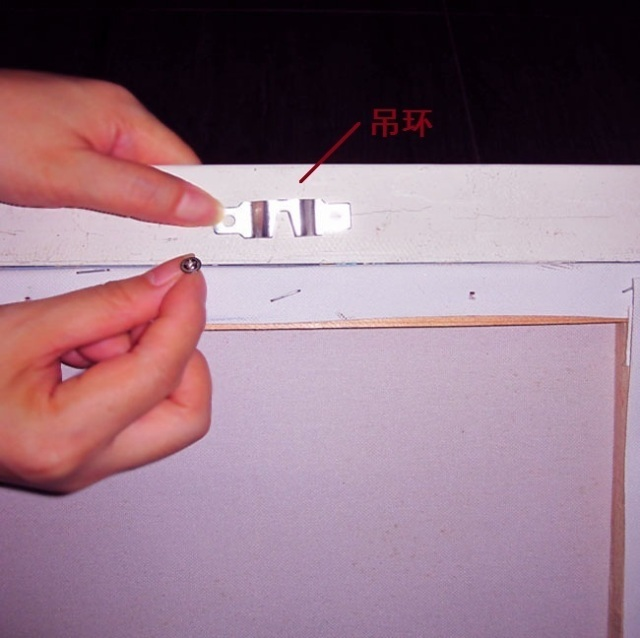▲ 图源：china.makepolo.com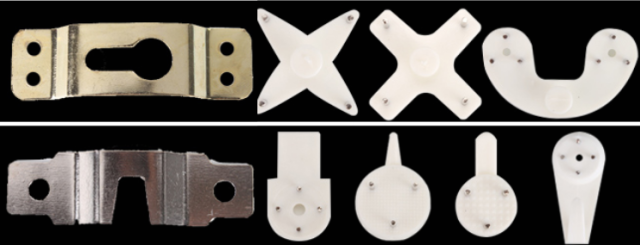▲ 不同的吊环搭配不同样式的无痕钉 图源： detail.tmall.com

另外也需要预估相框的重量，来选择无痕钉的型号大小。

它的最大的承重力能达到13公斤，应该是能够满足普通家庭挂画的需要的。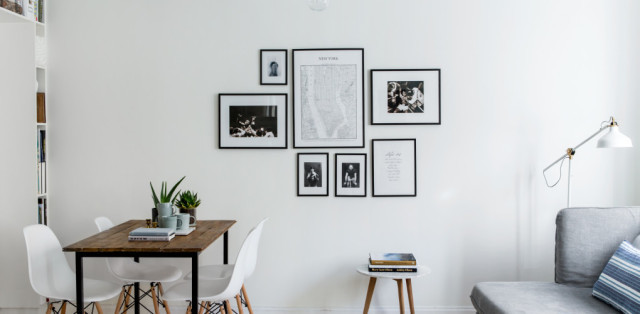▲ 图源： canvas.saatchiart.com

有了无痕钉之后，挂画就会变的非常容易。只要四步就可以将无痕钉固定在墙上。如果小伙伴们对横平竖直没概念，往往很难调整画框使它摆正，小小的气泡水平仪就能够解决这个问题。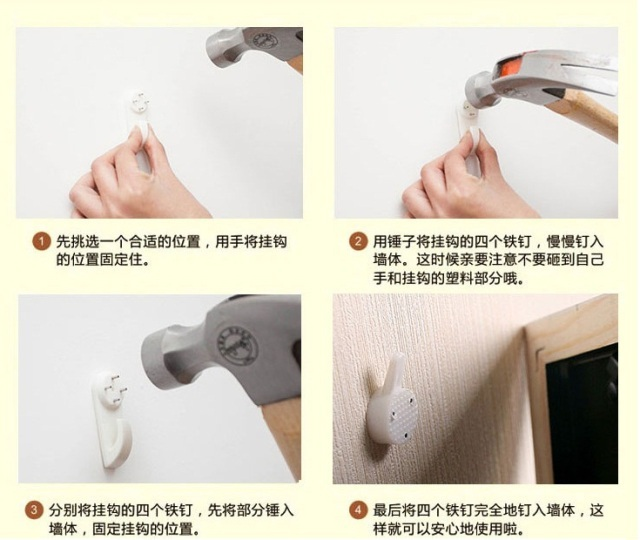▲ 图源：detail.tmall.com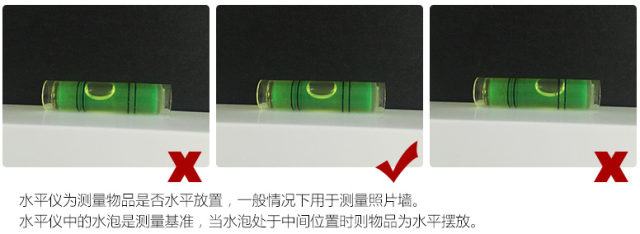▲ 购买无痕钉套餐里就有它 图源： detail.tmall.com

不过无痕钉在承重墙面、瓷砖和硅藻泥墙面都是不能用的，这一点大家也要记好哟。

▏粘钩为什么不行▕

很多小伙伴会觉得在墙上打孔多麻烦，不能使用粘钩吗？能，但不能挂重物。

如果想要在墙上悬挂的是艺术照、结婚照，那么大幅的尺寸，那么厚重的外框，放过粘钩吧，这是它生命不能承受之重。

作为一个有故事的女同学，东亭就亲眼看到菜刀从自家粘钩上掉落，所以答应我，不要尝试考验粘钩好吗？

而且粘钩对墙面的要求高，在壁纸、墙布或者有花纹的瓷砖，掉灰的墙面上都会贴不牢。▲ 图源： .nipic.com

▏蓝丁胶怎么样▕

蓝丁胶漂洋过海从土澳来到中国，以黏性高，操作简单，移除无痕三大特性迅速占领某宝墙上用胶的一席之地，而且它不！挑！墙！面！▲ 对蓝丁胶没概念的小伙伴见上图 图源： qianlijob.com

然而，蓝丁胶产品的质量良莠不齐，它的承重力有限。

而且靠近暖气的话，蓝丁胶的黏性会降低。即便没有暖气，蓝丁胶也是一种短期有效，但是超过一年就不怎么好使了的胶体。

所以想在墙上悬挂画框之类的物品，最好的选择还是无痕钉啦。

▏画摆在置物架上不行吗▕

看了很多家居美图，大家应该都能总结出了三大套路。

把画直接挂墙上，放墙面搁板上，摆地上。

把画挂墙上，一个小小的无痕钉就解决啦，而如果想把话放搁板上，则需要在墙上打孔，然后塞膨胀螺丝去固定搁板。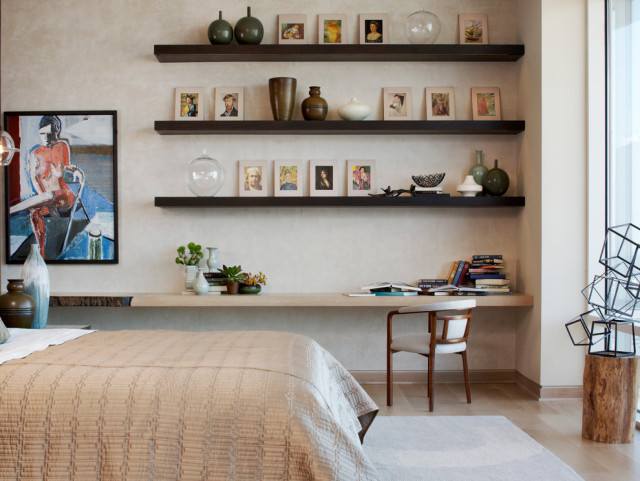▲ 图源：bukit.co

现在家里装修墙面挂物很少会使用钉子了。

除了置物隔板，厨卫挂杆的安装也都离不开膨胀螺丝。

要知道，厨卫作为杂物集中地，需要收纳的东西实在是太多。所以有个吊杆加挂篮的组合真的是超级棒。▲ 图源： ikea.com

常见的膨胀螺丝直径有6/8/10/12mm四种，最大的静止受力可达510公斤，承重力非常棒。

安装时要用和螺丝直径相同的钻头在墙上开孔，再塞入螺丝拧好就行了。

▲ 图源：china.makepolo.com

二

家人不让墙上打孔怎么办

▏墙上打孔也不是什么大事▕

很多人对于新装修的房子，都舍不得在墙面上打孔。

不打孔的理由听起来也很有道理，比如乳胶漆墙面打孔以后想家里换个布置，遗留下来的孔会非常难看啊；比如看见别人家瓷砖打孔结果开裂了很担心啊；比如听说承重墙不能打孔有危险啊。

那么墙面打孔真的不行吗？

根据规范，一般家庭墙面打孔只要避开钢筋和水电管道，孔的直径在1-25cm都是可以直接打的。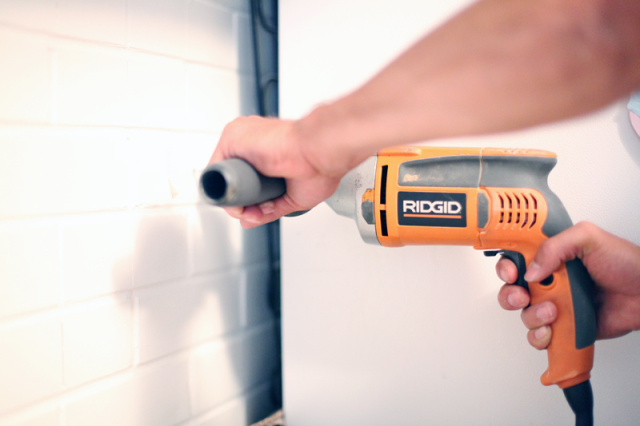▲ 图源： abeautifulmess.com

而且乳胶漆墙面挂幅画塞个膨胀螺丝留下的洞修补起来也完全不麻烦。

而瓷砖打孔开裂，很大的可能是师傅不够专业，或者小白自己买工具上手的结果，所以这个锅打孔不背。▲ 图源： contractortalk.com

其实父母辈抗拒墙上打孔也就是一个惯性认知，想改变并不难。

萌萌哒宇宙英雄就透露，自打她在家里墙上挂了一幅画之后，当初非常不乐意的爸妈也开始不亦乐乎的在墙上钉钉子挂物品。

你看，事情解决起来就这么简单。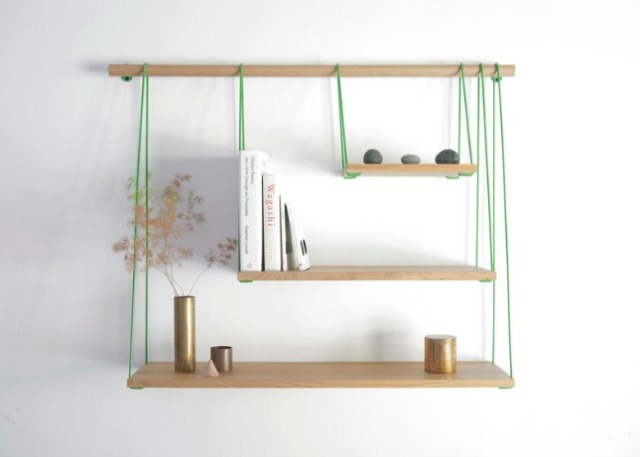▲ 图源：brit.co

三

墙面打孔的注意事项有哪些

既然我们是在家里力排众议才得到墙面打孔的机会，所以打孔时我们就要考虑好如何将墙面的破坏降低到最少。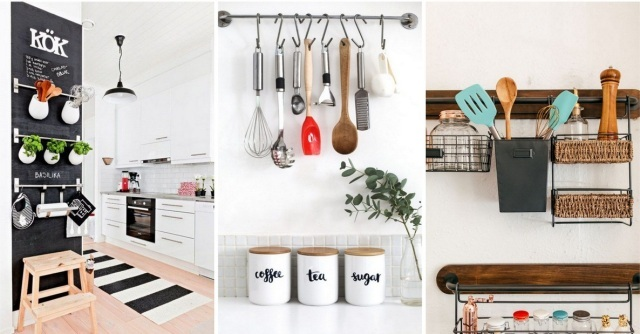▲ 图源：homesthetics.net

▏贴胶带▕

使用隐形钉时可以在确定好的位置上贴一段胶带，然后再用锤子把挂钩轻锤固定在墙面，这样可以防止钉子钉不牢，保护墙面不会有裂痕。

这一点也适用于瓷砖打孔。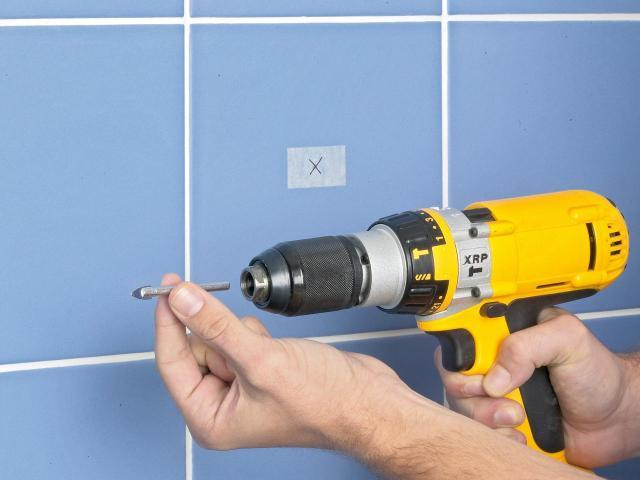▲ 图源：diynetwork.com

▏瓷砖不能空鼓▕

如果是在瓷砖上打孔，先要判断瓷砖是否空鼓，因为在空鼓的瓷砖上打孔，瓷砖一定会开裂的。

判断的方法很简单，用铁棒敲一敲，听声音，声音清脆就是空鼓了。

当然啦，瓷砖铺贴合格的话是不会出现这样的问题的。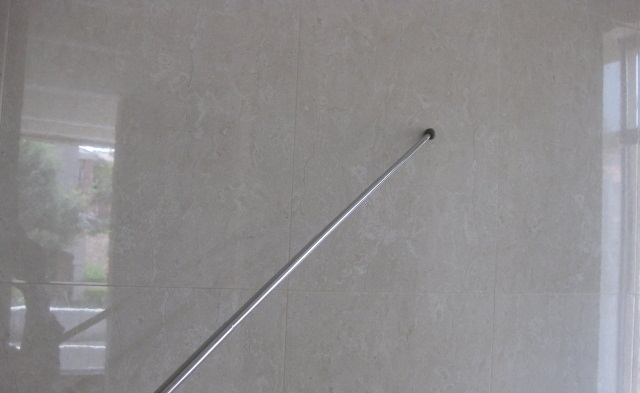▲ 图源：baike.zhaoshangbao.com

▏钻头要挑好▕

用钻头打孔时同样需要先贴好胶带，一般普通墙面打孔用冲击钻就行。

在瓷砖上打孔，方法有很多。

有经验的师傅会使用玻璃钻头，或者是冲击钻用合金钻头切换到无冲击模式，第三种是直接先拿钉子将瓷砖表面的釉面砸去，这样再钻孔才不会将瓷砖墙面打裂。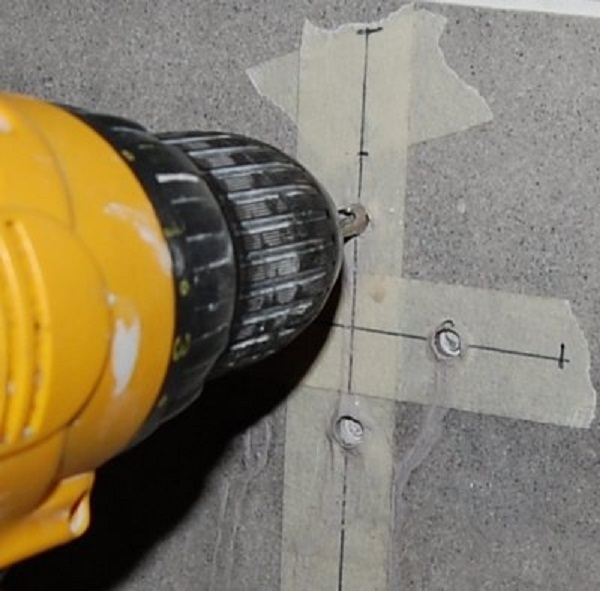▲ 图源： 365drills.com

▏小白不要亲自上▕

商家不提供安装服务的话，会把配套的螺丝，安装说明书都一起发过来，包括钻头的型号也会告知，所以有些小伙伴就会产生自己动手打孔的想法。

如果是新手小白，家里以后也很少会遇到打孔的情况的话，东亭觉得小伙伴们还是找专门的打孔师傅吧，更加专业省事。

而且比起动辄几百好一点要上千的电钻，打孔师傅一般收费只要几十块钱，不是更加经济实惠吗？

四

墙面修复

▏乳胶漆墙面修复怎么做▕

如果家里过了几年想要换个布局，之前墙上留下的冲击孔和钉痕就成了历史遗留问题。

但其实普通乳胶漆墙面修补起来也不难。

市面上有成品的腻子粉膏，又叫补墙膏，只要将它直接批刷在破损的墙面上，等干后用砂纸打磨，再刷上同色墙漆就搞定啦。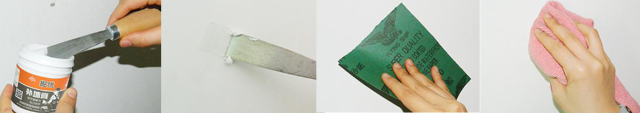▲ 图源：detail.tmall.com

▏瓷砖墙面修补呢▕

而瓷砖的修补就不那么容易了，如果不追求美观，可以用白水泥将孔堵上，表面再涂上白色防水的玻璃胶。

不愿意将就的话，就需要换掉打孔的瓷砖了。

当然，这个时候寻找同色瓷砖就会成为一个新的问题。

所以装修时，小伙伴们要记得，一定要预留几块多余的瓷砖哟。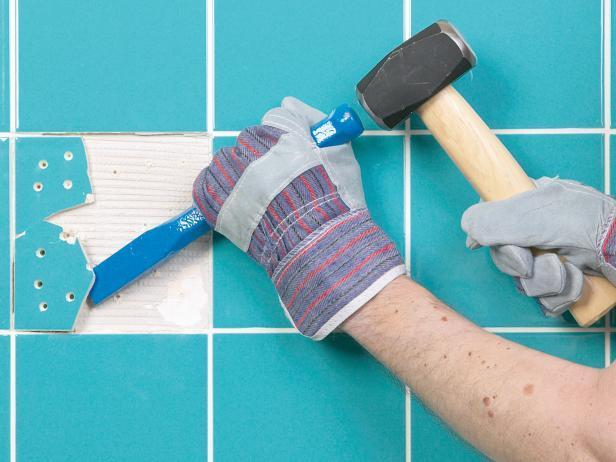▲ 图源： diynetwork.com

`声明：本文由入驻焦点开放平台的作者撰写，除焦点官方账号外，观点仅代表作者本人，不代表焦点立场错误信息举报电话： 400-099-0099，邮箱：jubao@vip.sohu.com，或点此进行意见反馈，或点此进行举报投诉。`A B C D E F G H J K L M N P Q R S T W X Y Z
A - B - C - D - E
• A
• 鞍山
• 安庆
• 安阳
• 安顺
• 安康
• 澳门
• B
• 北京
• 保定
• 包头
• 巴彦淖尔
• 本溪
• 蚌埠
• 亳州
• 滨州
• 北海
• 百色
• 巴中
• 毕节
• 保山
• 宝鸡
• 白银
• 巴州
• C
• 承德
• 沧州
• 长治
• 赤峰
• 朝阳
• 长春
• 常州
• 滁州
• 池州
• 长沙
• 常德
• 郴州
• 潮州
• 崇左
• 重庆
• 成都
• 楚雄
• 昌都
• 慈溪
• 常熟
• D
• 大同
• 大连
• 丹东
• 大庆
• 东营
• 德州
• 东莞
• 德阳
• 达州
• 大理
• 德宏
• 定西
• 儋州
• 东平
• E
• 鄂尔多斯
• 鄂州
• 恩施
F - G - H - I - J
• F
• 抚顺
• 阜新
• 阜阳
• 福州
• 抚州
• 佛山
• 防城港
• G
• 赣州
• 广州
• 桂林
• 贵港
• 广元
• 广安
• 贵阳
• 固原
• H
• 邯郸
• 衡水
• 呼和浩特
• 呼伦贝尔
• 葫芦岛
• 哈尔滨
• 黑河
• 淮安
• 杭州
• 湖州
• 合肥
• 淮南
• 淮北
• 黄山
• 菏泽
• 鹤壁
• 黄石
• 黄冈
• 衡阳
• 怀化
• 惠州
• 河源
• 贺州
• 河池
• 海口
• 红河
• 汉中
• 海东
• I
• J
• 晋中
• 锦州
• 吉林
• 鸡西
• 佳木斯
• 嘉兴
• 金华
• 景德镇
• 九江
• 吉安
• 济南
• 济宁
• 焦作
• 荆门
• 荆州
• 江门
• 揭阳
• 金昌
• 酒泉
• 嘉峪关
K - L - M - N - P
• K
• 开封
• 昆明
• 昆山
• L
• 廊坊
• 临汾
• 辽阳
• 连云港
• 丽水
• 六安
• 龙岩
• 莱芜
• 临沂
• 聊城
• 洛阳
• 漯河
• 娄底
• 柳州
• 来宾
• 泸州
• 乐山
• 六盘水
• 丽江
• 临沧
• 拉萨
• 林芝
• 兰州
• 陇南
• M
• 牡丹江
• 马鞍山
• 茂名
• 梅州
• 绵阳
• 眉山
• N
• 南京
• 南通
• 宁波
• 南平
• 宁德
• 南昌
• 南阳
• 南宁
• 内江
• 南充
• P
• 盘锦
• 莆田
• 平顶山
• 濮阳
• 攀枝花
• 普洱
• 平凉
Q - R - S - T - W
• Q
• 秦皇岛
• 齐齐哈尔
• 衢州
• 泉州
• 青岛
• 清远
• 钦州
• 黔南
• 曲靖
• 庆阳
• R
• 日照
• 日喀则
• S
• 石家庄
• 沈阳
• 双鸭山
• 绥化
• 上海
• 苏州
• 宿迁
• 绍兴
• 宿州
• 三明
• 上饶
• 三门峡
• 商丘
• 十堰
• 随州
• 邵阳
• 韶关
• 深圳
• 汕头
• 汕尾
• 三亚
• 三沙
• 遂宁
• 山南
• 商洛
• 石嘴山
• T
• 天津
• 唐山
• 太原
• 通辽
• 铁岭
• 泰州
• 台州
• 铜陵
• 泰安
• 铜仁
• 铜川
• 天水
• 天门
• W
• 乌海
• 乌兰察布
• 无锡
• 温州
• 芜湖
• 潍坊
• 威海
• 武汉
• 梧州
• 渭南
• 武威
• 吴忠
• 乌鲁木齐
X - Y - Z
• X
• 邢台
• 徐州
• 宣城
• 厦门
• 新乡
• 许昌
• 信阳
• 襄阳
• 孝感
• 咸宁
• 湘潭
• 湘西
• 西双版纳
• 西安
• 咸阳
• 西宁
• 仙桃
• 西昌
• Y
• 运城
• 营口
• 盐城
• 扬州
• 鹰潭
• 宜春
• 烟台
• 宜昌
• 岳阳
• 益阳
• 永州
• 阳江
• 云浮
• 玉林
• 宜宾
• 雅安
• 玉溪
• 延安
• 榆林
• 银川
• Z
• 张家口
• 镇江
• 舟山
• 漳州
• 淄博
• 枣庄
• 郑州
• 周口
• 驻马店
• 株洲
• 张家界
• 珠海
• 湛江
• 肇庆
• 中山
• 自贡
• 资阳
• 遵义
• 昭通
• 张掖
• 中卫

1室1厅1厨1卫1阳台

1
2
3
4
5

0
1
2

1

1

0
1
2
3报名成功，资料已提交审核A B C D E F G H J K L M N P Q R S T W X Y Z
A - B - C - D - E
• A
• 鞍山
• 安庆
• 安阳
• 安顺
• 安康
• 澳门
• B
• 北京
• 保定
• 包头
• 巴彦淖尔
• 本溪
• 蚌埠
• 亳州
• 滨州
• 北海
• 百色
• 巴中
• 毕节
• 保山
• 宝鸡
• 白银
• 巴州
• C
• 承德
• 沧州
• 长治
• 赤峰
• 朝阳
• 长春
• 常州
• 滁州
• 池州
• 长沙
• 常德
• 郴州
• 潮州
• 崇左
• 重庆
• 成都
• 楚雄
• 昌都
• 慈溪
• 常熟
• D
• 大同
• 大连
• 丹东
• 大庆
• 东营
• 德州
• 东莞
• 德阳
• 达州
• 大理
• 德宏
• 定西
• 儋州
• 东平
• E
• 鄂尔多斯
• 鄂州
• 恩施
F - G - H - I - J
• F
• 抚顺
• 阜新
• 阜阳
• 福州
• 抚州
• 佛山
• 防城港
• G
• 赣州
• 广州
• 桂林
• 贵港
• 广元
• 广安
• 贵阳
• 固原
• H
• 邯郸
• 衡水
• 呼和浩特
• 呼伦贝尔
• 葫芦岛
• 哈尔滨
• 黑河
• 淮安
• 杭州
• 湖州
• 合肥
• 淮南
• 淮北
• 黄山
• 菏泽
• 鹤壁
• 黄石
• 黄冈
• 衡阳
• 怀化
• 惠州
• 河源
• 贺州
• 河池
• 海口
• 红河
• 汉中
• 海东
• I
• J
• 晋中
• 锦州
• 吉林
• 鸡西
• 佳木斯
• 嘉兴
• 金华
• 景德镇
• 九江
• 吉安
• 济南
• 济宁
• 焦作
• 荆门
• 荆州
• 江门
• 揭阳
• 金昌
• 酒泉
• 嘉峪关
K - L - M - N - P
• K
• 开封
• 昆明
• 昆山
• L
• 廊坊
• 临汾
• 辽阳
• 连云港
• 丽水
• 六安
• 龙岩
• 莱芜
• 临沂
• 聊城
• 洛阳
• 漯河
• 娄底
• 柳州
• 来宾
• 泸州
• 乐山
• 六盘水
• 丽江
• 临沧
• 拉萨
• 林芝
• 兰州
• 陇南
• M
• 牡丹江
• 马鞍山
• 茂名
• 梅州
• 绵阳
• 眉山
• N
• 南京
• 南通
• 宁波
• 南平
• 宁德
• 南昌
• 南阳
• 南宁
• 内江
• 南充
• P
• 盘锦
• 莆田
• 平顶山
• 濮阳
• 攀枝花
• 普洱
• 平凉
Q - R - S - T - W
• Q
• 秦皇岛
• 齐齐哈尔
• 衢州
• 泉州
• 青岛
• 清远
• 钦州
• 黔南
• 曲靖
• 庆阳
• R
• 日照
• 日喀则
• S
• 石家庄
• 沈阳
• 双鸭山
• 绥化
• 上海
• 苏州
• 宿迁
• 绍兴
• 宿州
• 三明
• 上饶
• 三门峡
• 商丘
• 十堰
• 随州
• 邵阳
• 韶关
• 深圳
• 汕头
• 汕尾
• 三亚
• 三沙
• 遂宁
• 山南
• 商洛
• 石嘴山
• T
• 天津
• 唐山
• 太原
• 通辽
• 铁岭
• 泰州
• 台州
• 铜陵
• 泰安
• 铜仁
• 铜川
• 天水
• 天门
• W
• 乌海
• 乌兰察布
• 无锡
• 温州
• 芜湖
• 潍坊
• 威海
• 武汉
• 梧州
• 渭南
• 武威
• 吴忠
• 乌鲁木齐
X - Y - Z
• X
• 邢台
• 徐州
• 宣城
• 厦门
• 新乡
• 许昌
• 信阳
• 襄阳
• 孝感
• 咸宁
• 湘潭
• 湘西
• 西双版纳
• 西安
• 咸阳
• 西宁
• 仙桃
• 西昌
• Y
• 运城
• 营口
• 盐城
• 扬州
• 鹰潭
• 宜春
• 烟台
• 宜昌
• 岳阳
• 益阳
• 永州
• 阳江
• 云浮
• 玉林
• 宜宾
• 雅安
• 玉溪
• 延安
• 榆林
• 银川
• Z
• 张家口
• 镇江
• 舟山
• 漳州
• 淄博
• 枣庄
• 郑州
• 周口
• 驻马店
• 株洲
• 张家界
• 珠海
• 湛江
• 肇庆
• 中山
• 自贡
• 资阳
• 遵义
• 昭通
• 张掖
• 中卫• 手机• 分享
• 设计
免费设计
• 计算器
装修计算器
• 入驻
合作入驻
• 联系
联系我们
• 置顶
返回顶部